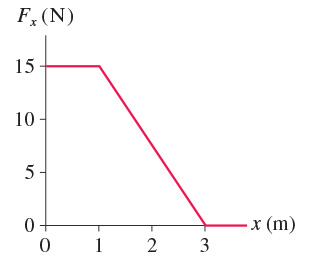# Problem: A 400 g particle moving along the x-axis experiences the force shown in the figure. The particle's velocity is 9.0 m/s at x = 0 m. What is its velocity at x = 3 m?

🤓 Based on our data, we think this question is relevant for Professor Jarrio's class at GT.

###### Problem Details
A 400 g particle moving along the x-axis experiences the force shown in the figure. The particle's velocity is 9.0 m/s at x = 0 m. What is its velocity at x = 3 m?